Browse by Topic
Related Topics

# Star Light, Star Bright: Stellar Aperture Photometry with the Wolfram Language

Stellar CCD aperture photometry is the technique of extracting information about the brightness of stars from a series of images collected over time. The light curve of a variable star can reveal useful information about the physics of the star, including a measure of its intrinsic brightness. Light curve analysis can yield information about eclipsing binary systems, and also lead to exoplanet discoveries when a planet alters the brightness of a star by crossing its disk as viewed from Earth.

In CCD photometry, we want to be able to determine a measure of the amount of radiation coming from a given star arriving on our CCD detector. Plotted as a function of time, this measurement can reveal important information about the star or star system.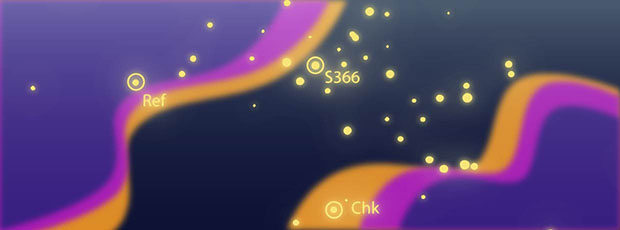The Wolfram Language is an ideal tool for extracting and analyzing this data because it combines image processing, data reduction, plotting, curve fitting, file import and astronomical data into one package. In this post, I will review some of the basic techniques of stellar aperture CCD photometry using the Wolfram Language.

## Cepheid Variables

With a Cepheid variable star, there is a strong correlation between the star’s light curve and the star’s intrinsic brightness, or absolute magnitude. This period-luminosity relationship, first published in 1912, is called Leavitt’s law, after Henrietta Swan Leavitt, based on her work at the Harvard College Observatory.

Leavitt worked as a “human computer” at the observatory, manually extracting observation data from glass plates, for which she was paid \$0.30 per hour (eventually).

For Population I Cepheids, Leavitt’s law relates the Log of the star’s period (in days) to the mean absolute magnitude: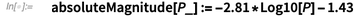✕ `absoluteMagnitude[P_] := -2.81*Log10[P] - 1.43`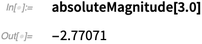✕ `absoluteMagnitude[3.0]`

Here is a log-linear plot of the data: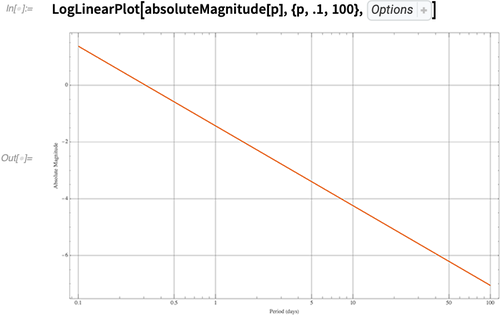✕ ```LogLinearPlot[absoluteMagnitude[p], {p, .1, 100}, { PlotTheme -> "Scientific", GridLines -> Automatic, FrameLabel -> {"Period (days)", "Absolute Magnitude"}, ImageSize -> 800}]```

Since the observed apparent magnitude diminishes the further the star is from Earth, the distance (in parsecs) can be determined from the period and the apparent magnitude: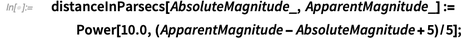✕ ```distanceInParsecs[AbsoluteMagnitude_, ApparentMagnitude_] := Power[10.0, (ApparentMagnitude - AbsoluteMagnitude + 5)/5];```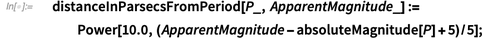✕ ```distanceInParsecsFromPeriod[P_, ApparentMagnitude_] := Power[10.0, (ApparentMagnitude - absoluteMagnitude[P] + 5)/5];```

## Hubble’s Discovery

In 1924, Edwin Hubble identified a Cepheid variable in what was then known as the “Andromeda Nebula.”

By extracting its absolute magnitude from the Cepheid’s light curve and comparing it to the observed magnitude, he was able to show the distance to the star was millions of light years and prove that it was part of an entirely separate galaxy—thus vastly expanding the size of the known universe.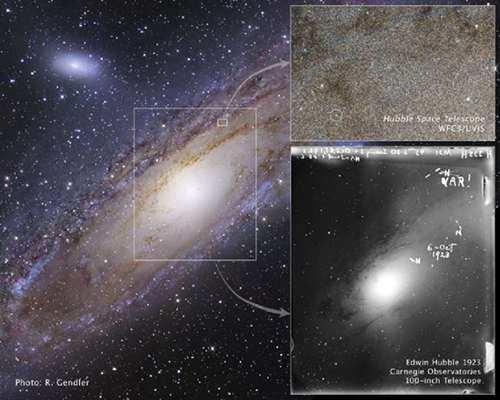## Some Assumptions

We must make some assumptions about the images in order to compare them against each other. We calibrate the image frames by doing the following:

• Subtracting dark frames (removes thermal noise)
• Subtracting flat frames (removes illumination variation)
• Aligning image frames (allows photometric comparison across multiple images)

These steps allow us to compare different stars across a CCD field and get accurate magnitude comparisons. Bias noise associated with reading the CCD array is automatically subtracted out by the main photometry computation.

## Technique

First, divide the area around our star into three regions: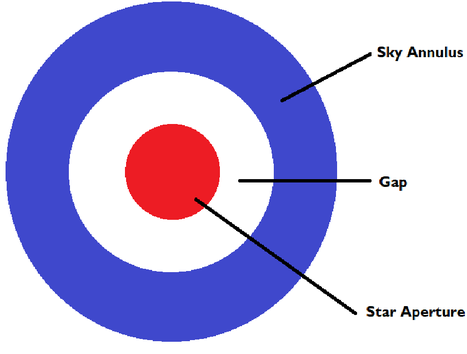The center disk isolates photons coming from the star. The next ring is actually a gap, an empty space separating the star aperture from the sky annulus. The third and outermost ring represents the sky annulus, which isolates sky background photons.

Simple image processing techniques can split apart these sections of the image around the target star so that we can total up the image values (and thus the photon count ) in each section. The way we do this is to generate a disk or an annular graphic in the color white, with everything else being black. This mask image is then multiplied onto the target image (since an image is just an array of numeric data values), which results in an image with everything black (value = 0.0) except for the area where we want to total up the data.

We can use the Wolfram Language to generate these disk and annular masks to isolate the regions on the raster: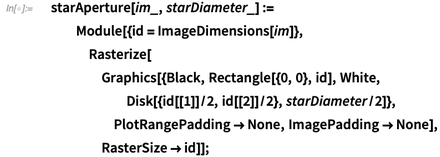✕ ```starAperture[im_, starDiameter_] := Module[{id = ImageDimensions[im]}, Rasterize[ Graphics[{Black, Rectangle[{0, 0}, id], White, Disk[{id[]/2, id[]/2}, starDiameter/2]}, PlotRangePadding -> None, ImagePadding -> None], RasterSize -> id]];```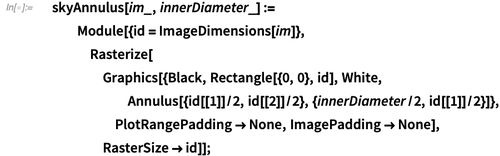✕ ```skyAnnulus[im_, innerDiameter_] := Module[{id = ImageDimensions[im]}, Rasterize[ Graphics[{Black, Rectangle[{0, 0}, id], White, Annulus[{id[]/2, id[]/2}, {innerDiameter/2, id[]/2}]}, PlotRangePadding -> None, ImagePadding -> None], RasterSize -> id]];```

We can apply this to any image. For this example, in the spirit of Edward Weston, let’s use these masks to isolate parts of an image of some peppers: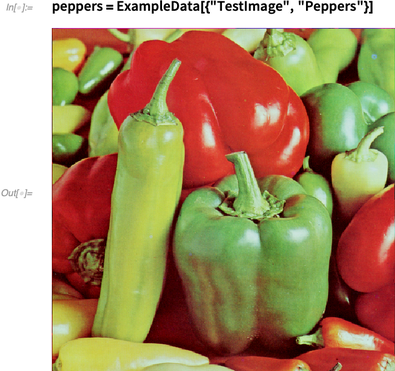✕ `peppers = ExampleData[{"TestImage", "Peppers"}]`

We can find the star aperture of the peppers: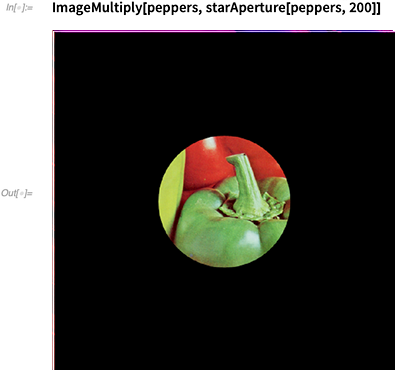✕ `ImageMultiply[peppers, starAperture[peppers, 200]]`

And here we find the “sky annulus” of the peppers image: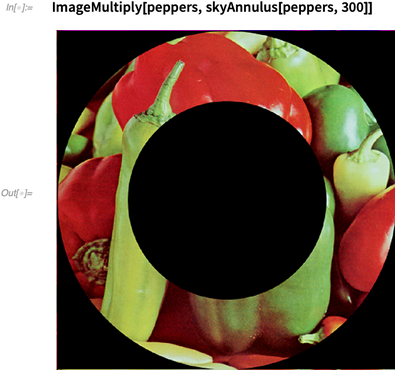✕ `ImageMultiply[peppers, skyAnnulus[peppers, 300]]`

We can see that the remaining ring of peppers is the “sky annulus,” with the black circle in the center accounting for the star aperture and gap.

## Computing Star Brightness

To compute the total star brightness, we subtract the sky background. First, we isolate the star of interest from the full CCD frame: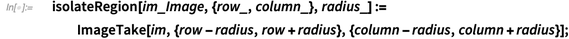✕ ```isolateRegion[im_Image, {row_, column_}, radius_] := ImageTake[ im, {row - radius, row + radius}, {column - radius, column + radius}];```

With the star isolated, we subtract the sky background and compute the total signal coming from the star: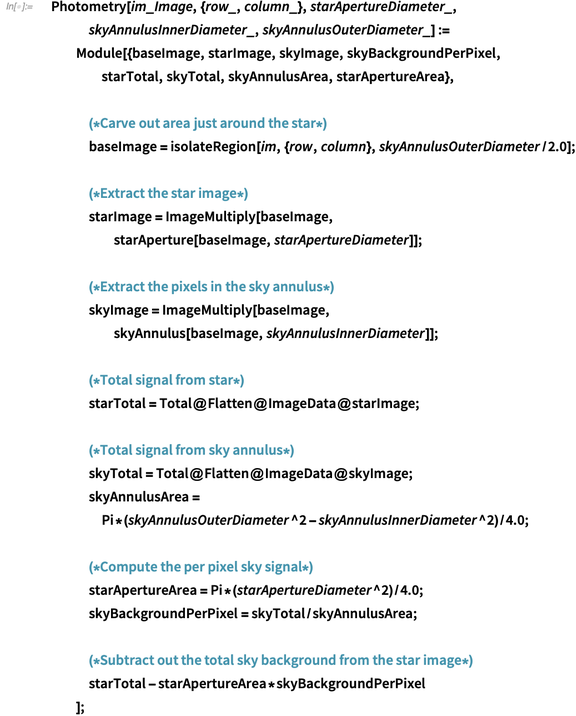✕ ```Photometry[im_Image, {row_, column_}, starApertureDiameter_, skyAnnulusInnerDiameter_, skyAnnulusOuterDiameter_] := Module[{baseImage, starImage, skyImage, skyBackgroundPerPixel, starTotal, skyTotal, skyAnnulusArea, starApertureArea}, (*Carve out area just around the star*) baseImage = isolateRegion[im, {row, column}, skyAnnulusOuterDiameter/2.0]; (*Extract the star image*) starImage = ImageMultiply[baseImage, starAperture[baseImage, starApertureDiameter]]; (*Extract the pixels in the sky annulus*) skyImage = ImageMultiply[baseImage, skyAnnulus[baseImage, skyAnnulusInnerDiameter]]; (*Total signal from star*) starTotal = Total@Flatten@ImageData@starImage; (*Total signal from sky annulus*) skyTotal = Total@Flatten@ImageData@skyImage; skyAnnulusArea = Pi*(skyAnnulusOuterDiameter^2 - skyAnnulusInnerDiameter^2)/4.0; (*Compute the per pixel sky signal*) starApertureArea = Pi*(starApertureDiameter^2)/4.0; skyBackgroundPerPixel = skyTotal/skyAnnulusArea; (*Subtract out the total sky background from the star image*) starTotal - starApertureArea*skyBackgroundPerPixel ];```

## Determining the Time the Image Was Taken

The goal in photometry is to obtain a series of brightness values as a function of time. For this, we need an accurate observation time for each value.

Astronomical image data is traditionally collected in the form of FITS (Flexible Image Transport System) data files, which Mathematica can easily import. Each FITS file, in addition to image data, can contain a wealth of metadata describing the details of the observation associated with the image, including the time and date the image was taken. For convenience, we are going to represent the image time in the form of a Julian date.

A Julian date is a real number representing the number of days that have transpired since noon Universal Time on January 1, 4713 BC (on the Julian calendar). Many FITS files contain the observation time as a Julian date. Even if a FITS file doesn’t contain an explicit Julian date, we can still derive that value from other time information that is often found in the FITS metadata.

For convenience, let’s use an Association to bundle together the FITS image and the metadata into a FITS object: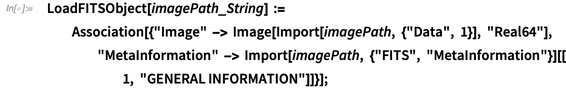✕ ```LoadFITSObject[imagePath_String] := Association[{"Image" -> Image[Import[imagePath, {"Data", 1}], "Real64"], "MetaInformation" -> Import[imagePath, {"FITS", "MetaInformation"}][[1, "GENERAL INFORMATION"]]}]; ```

Unfortunately, there are several ways that the observation date can be represented in the metadata of FITS files, so we will need to determine which method we want to use for the remainder of our calculations: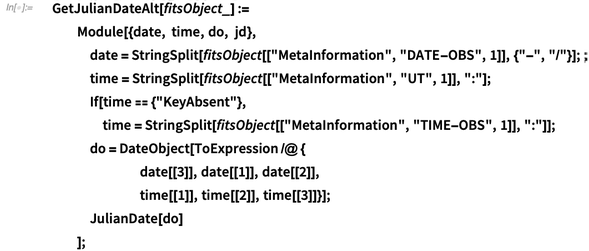✕ ```GetJulianDateAlt[fitsObject_] := Module[{date, time, do, jd}, date = StringSplit[ fitsObject[["MetaInformation", "DATE-OBS", 1]], {"-", "/"}]; time = StringSplit[fitsObject[["MetaInformation", "UT", 1]], ":"]; If[time == {"KeyAbsent"}, time = StringSplit[fitsObject[["MetaInformation", "TIME-OBS", 1]], ":"]]; do = DateObject[ToExpression /@ { date[], date[], date[], time[], time[], time[]}]; JulianDate[do] ];```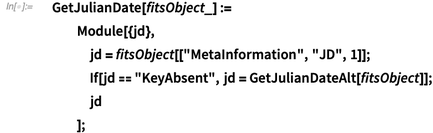✕ ```GetJulianDate[fitsObject_] := Module[{jd}, jd = fitsObject[["MetaInformation", "JD", 1]]; If[jd == "KeyAbsent", jd = GetJulianDateAlt[fitsObject]]; jd ];```

## Extracting Brightness

Now we can extract brightness as a function of time by using a (non-varying) comparison star of a known magnitude: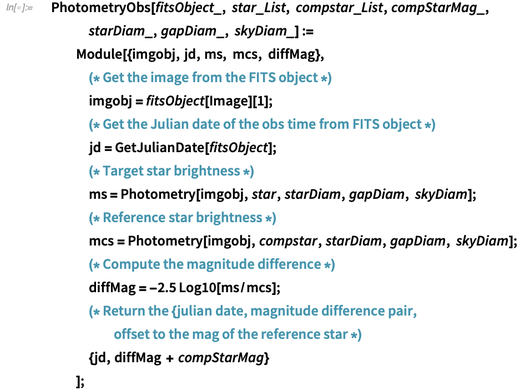✕ ```PhotometryObs[fitsObject_, star_List, compstar_List, compStarMag_, starDiam_, gapDiam_, skyDiam_] := Module[{imgobj, jd, ms, mcs, diffMag}, (* Get the image from the FITS object *) imgobj = fitsObject[["Image"]]; (* Get the Julian date of the obs time from FITS object *) jd = GetJulianDate[fitsObject]; (* Target star brightness *) ms = Photometry[imgobj, star, starDiam, gapDiam, skyDiam]; (* Reference star brightness *) mcs = Photometry[imgobj, compstar, starDiam, gapDiam, skyDiam]; (* Compute the magnitude difference *) diffMag = -2.5 Log10[ms/mcs]; (* Return the {julian date, magnitude difference pair, offset to the mag of the reference star *) {jd, diffMag + compStarMag} ];```

## Cepheid Variable Light Curve

We can finally put our code to the test by analyzing a Cepheid variable light curve, a synthetic star field. For this example, we are using a set of synthetic star fields provided as teaching examples from this website. Select “Guest” at the prompt, and download all the FITS files from the S366 directory into a local folder for processing. You can also download the set here.

First we form a list of the names of the FITS files that contain our data: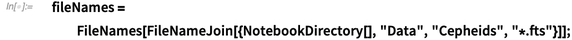✕ ```fileNames = FileNames[ FileNameJoin[{NotebookDirectory[], "Data", "Cepheids", "*.fts"}]];```

Then load “bundled” FITS objects by mapping the LoadFITSObject function onto this list: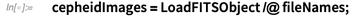✕ `cepheidImages = LoadFITSObject /@ fileNames;`

Thankfully, we have a star chart provided with this set that we can Import: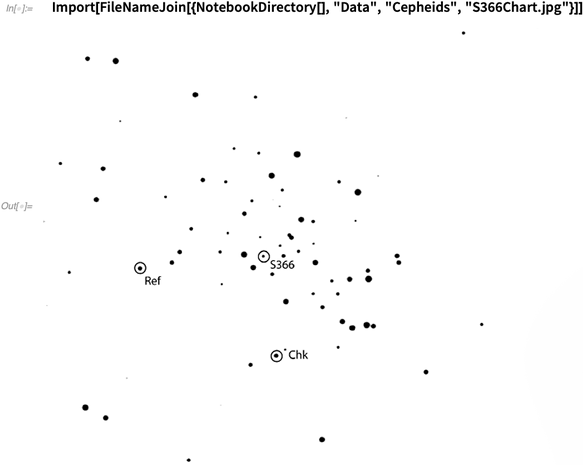✕ ```Import[FileNameJoin[{NotebookDirectory[], "Data", "Cepheids", "S366Chart.jpg"}]]```

Load the first image so we can locate the Cepheid on it: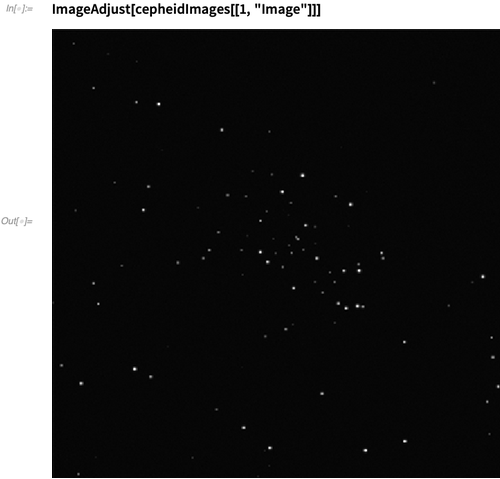✕ `ImageAdjust[cepheidImages[[1, "Image"]]]`

It is easy to locate the row and column of the target star by clicking the image of the star field in the notebook and using editing features to examine the star. Once we have the {row, column} coordinates of the star and a reference star on the image, we can extract the photometric data as a function of time, adjusted to the magnitude of the reference star (here taken to be 10.0).

From the examination of the image, we use a star diameter of 6 pixels, the outer gap diameter of 8 pixels and the sky annulus outer diameter of 20 pixels. We can then get the list of apparent magnitudes sorted by their Julian dates by mapping PhotometryObs onto the list of FITS objects and then sorting: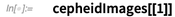✕ `cepheidImages[]`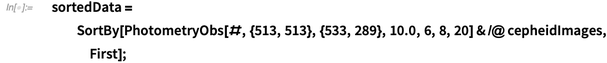✕ ```sortedData = SortBy[PhotometryObs[#, {513, 513}, {533, 289}, 10.0, 6, 8, 20] & /@ cepheidImages, First];```

Our data points are of the form {Julian Date, Apparent Magnitude}. Let’s retrieve the first data point: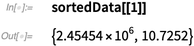✕ `sortedData[]`

We can plot our data points to see if there are any visible patterns. Here we plot the Cepheid light curve, starting from the earliest observation: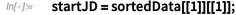✕ `startJD = sortedData[[1, 1]];`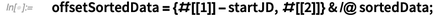✕ `offsetSortedData = {#[] - startJD, #[]} & /@ sortedData;`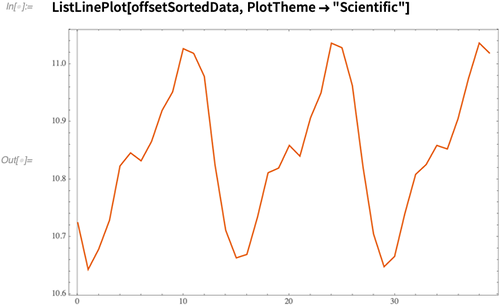✕ `ListLinePlot[offsetSortedData, PlotTheme -> "Scientific"]`

## Analyzing Data

After establishing our data points, our next valuable step is to analyze the data. Let’s try a simple fit to a Sin[] function to establish the period of the Cepheid’s cycle: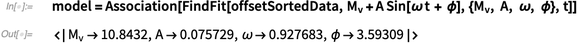✕ ```model = Association[ FindFit[offsetSortedData, Subscript[M, v] + A Sin[\[Omega] t + \[Phi]], {Subscript[M, v], A, \[Omega], \[Phi]}, t]]```

Here,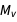is the apparent visual magnitude. However, we can do better. FindFit isn’t the most accurate way to determine the frequency parameter ω when fitting to periodic data. For periodic data, a fast Fourier transform (FFT) is a traditional method that can be used to convert time series data to frequency space; however, this technique requires data points that are regularly spaced. Unfortunately, photometric data isn’t usually of this type, because observations may be spaced over days, weeks or longer.

The best way to analyze data like this is to use IrregularPeriodogram, which is available in the Wolfram Function Repository. I’m not going to go into the math behind IrregularPeriodogram here, but if you’re interested, you can refer to the references cited at the end of this article. IrregularPeriodogram grew out of the needs of the astronomical community, which frequently needs to analyze data from non-regularly-spaced observations.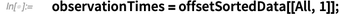✕ `observationTimes = offsetSortedData[[All, 1]];`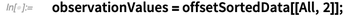✕ `observationValues = offsetSortedData[[All, 2]];`

IrregularPeriodogram needs the data to be zero offset from the mean: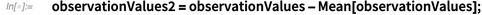✕ `observationValues2 = observationValues - Mean[observationValues];`

Now we can call IrregularPeriodogram from the Function Repository and plot the results: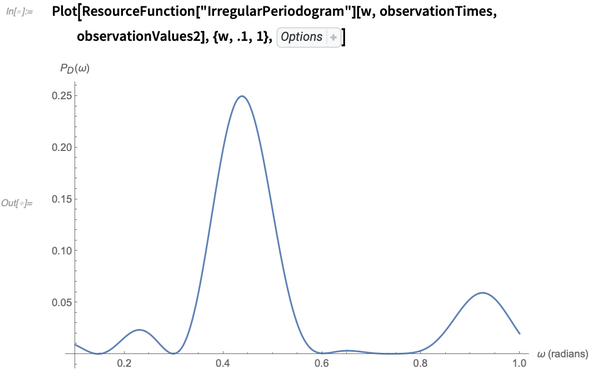✕ ```Plot[ResourceFunction["IrregularPeriodogram"][w, observationTimes, observationValues2], {w, .1, 1}, PlotPoints -> 300, PlotRange -> All, AxesLabel -> {"\[Omega] (radians)", "\!\(\*SubscriptBox[\(P\),\(D\)]\)(\[Omega])"}, ImageSize -> 800]```

This shows a strong peak near .4 radians, which corresponds to the fundamental angular frequency of the data. Numerically, this frequency can be determined by NArgMax: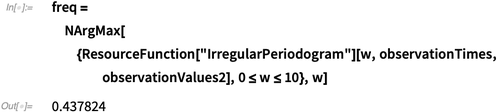✕ ```freq = NArgMax[{ResourceFunction["IrregularPeriodogram"][w, observationTimes, observationValues2], 0 <= w <= 10}, w]```

The period that corresponds to the lowest-order frequency is the period of the variable star in days: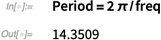✕ `Period = 2 \[Pi]/freq`

Using our distance-period relationship and the apparent visual magnitude of the star, we can then compute the distance in parsecs: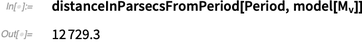✕ `distanceInParsecsFromPeriod[Period, model[Subscript[M, v]]]`

## Further into the Stars

For those who’d like to trek a little further into stellar aperture photometry, you can check out the eclipsing binary star BU Vulpeculae from the same University of Iowa website. I hope you’ve enjoyed this post, and that you’ll find it useful for your own exploration of the stars—see you out there!

Clarke, D. “String/Rope Length Methods Using the Lafler–Kinman Statistic.” Astronomy &
Astrophysics
. 386 (2002): 763–74.

Deeming, T. J. “Fourier Analysis with Unequally Spaced Data.” Astrophysics and Space Science. 36.1
(1975): 137–58.

Lafler, J. and T. D. Kinman. “An RR Lyrae Star Survey with the Lick 20-Inch Astrograph  II. The
Calculation of RR Lyrae Periods by Electronic Computer.” Astrophysical Journal Supplement. 11
(1965): 216.

Lomb, N. R. “Least-Squares Frequency Analysis of Unequally Spaced Data.”  Astrophysics and Space
Science
39 (1976): 447–62.

Scargle, J. D. “Studies in Astronomical Time Series Analysis. II Statistical Aspects of  Spectral
Analysis of Unevenly Spaced Data.” The Astrophysical Journal. 263 (1982): 835–53.

 Get full access to the latest Wolfram Language functionality with a Mathematica 12 or Wolfram|One trial.